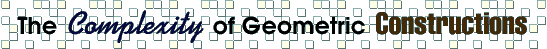by François Labelle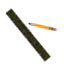This page is about efficient constructions with straight-edge and collapsing compass (the geometric tools of Euclid). The question of what can and cannot be constructed with this model of computation is basically answered by Galois Theory. However, given that a construction is possible, there's not much to be found on the minimum number of steps required, which incidentally is the subject of this document.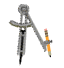### Our Model

 Some points in the plane are given to start with. These points are said to be constructed. Given two constructed points, the straight-edge can be used to draw an (infinite) line through them. Given two constructed points, the collapsing compass can be used to draw a circle with center at one point and passing through the other. The points of intersection of lines and circles are considered to be constructed. Java Applet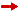more on the model

### Measure of Complexity

The complexity of a construction is defined to be the number of drawing operations (lines and circles) that are performed. Note that other complexity measures have been proposed.more on complexity measures

### Best Known Constructions

The main feature of this web page is a Java applet in which you can view the "best" solution (that I know of) to a variety of problems. Some solutions are just amazing!

You can also use the applet to try to solve one of the problems yourself. If you happen to beat the best known solution, tell me your solution and it will replace the old one. It's an open challenge!

Choose an applet size to go to the applet page:small applet: 580 x 320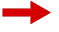large applet: 850 x 520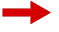arbitrary size: x

Euclid

Constructions
Impossible (in our model)
• Doubling the Cube (or constructing the number 21/3)
• Trisecting an Angle (in particular, constructing the number cos 20o)
• Squaring the Circle (or constructing the number Pi)
• Constructing a Regular Heptagon (or constructing the number cos 360o/7)paper references

This page is presented to Godfried Toussaint as a Web project for the Computational Geometry course 308-507A. Other student projects can be found here.
page created: December 5, 1997
last updated: June 7, 2014